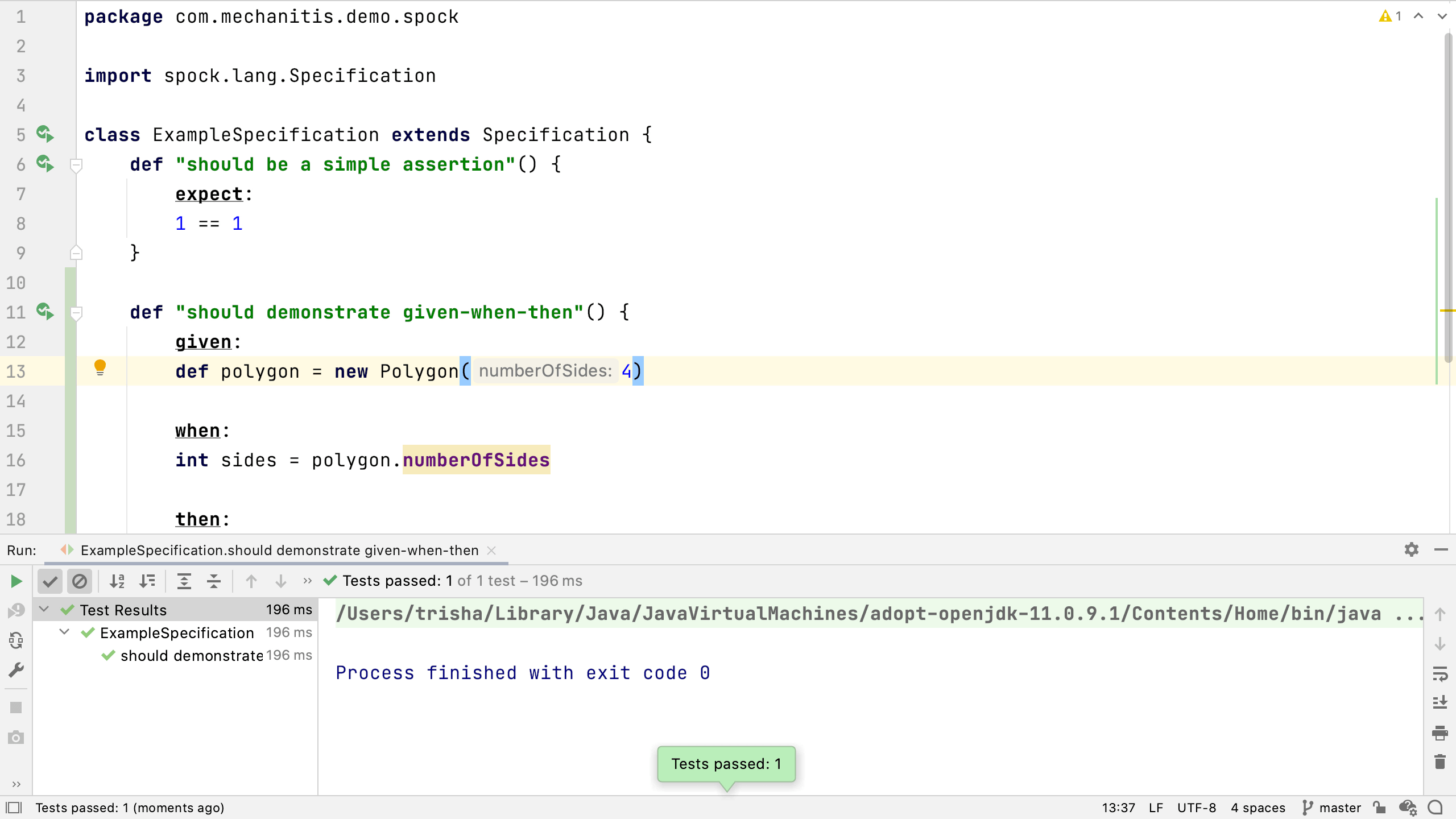## Given, When and Then

### See how Spock tests are usually structured.

One of the most powerful features of Spock is how descriptive it is. It's not just for testing code, it's for describing and documenting expected behaviour.

In our last test, we used the `expect` label to indicate a simple expectation. Spock supports a number of different labels for test blocks. My personal favourite set of labels is `given`, `when`, `then`. Let's create a new test method:

``def "should demonstrate given-when-then"() {    given:    def polygon = new Polygon(4)    when:    int sides = polygon.numberOfSides    then:    sides == 4}``

You'll also need to create a Java Polygon class in `src/main/java`. We can use Spock to test Java or Groovy code.

``public class Polygon {    private final int numberOfSides;    public Polygon(int numberOfSides) {        this.numberOfSides = numberOfSides;    }}``

Back in the test, you can see the `given` label is used to indicate that this part of the method is setting up the required conditions. This test needs an instance of `Polygon` with four sides.

``given:def polygon = new Polygon(4)``

Next, we say `when`. This is the thing we're actually testing, the behaviour we're trying to check. This test makes sure the `numberOfSides` has been correctly set and that we can get it from the class.

``when:int sides = polygon.numberOfSides``

Finally, we say `then`. This section checks all the conditions we expect to have been met. This test checks that the number of sides is four, the original number of sides passed in to the constructor.

``then:sides == 4``

You can read a given/when/then test like this:

• given that I have a Polygon with four sides,
• when we get the number of sides
• then that number of sides should be equal to four

Run this test to see if it passes. You should get an output like:Using these labels might look like it's doubling the number of lines of code for a test method, but remember that tests often have many more lines of code in them, and grouping them in this way helps describe what's happening.

In this step, we covered some tips on writing tests using Spock. Now you know how to use labels to define the blocks of your tests